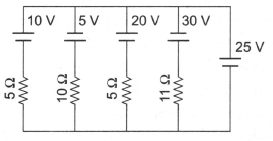Find the power supplied by 20 V cell in the figure shown.

# Find the power supplied by 20 V cell in the figure shown.1. A

$-10\text{\hspace{0.17em}\hspace{0.17em}}W$

2. B

20 W

3. C

$-20\text{\hspace{0.17em}\hspace{0.17em}}W$

4. D

10 W

Register to Get Free Mock Test and Study Material

+91

Verify OTP Code (required)

### Solution:

Taking point A as reference potential and its potential to be 0 : I = 20 A

Power supplied by 20V cell $=-20\text{\hspace{0.17em}\hspace{0.17em}}×\text{\hspace{0.17em}\hspace{0.17em}}1=-20\text{\hspace{0.17em}\hspace{0.17em}}W$Register to Get Free Mock Test and Study Material

+91

Verify OTP Code (required)